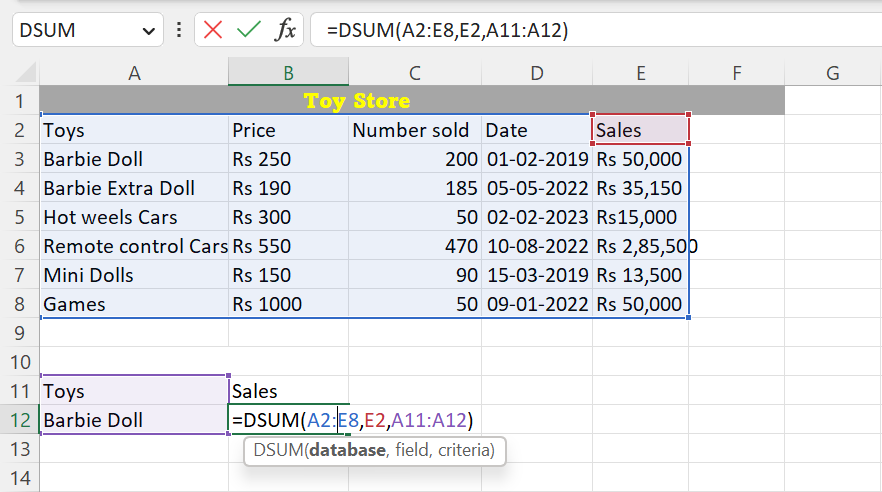top of page
Search

# How to use DSUM function in Excel

Updated: Mar 17

DSUM is an abbreviation for Database Sum, and it is used to sum values that meet specific criteria in a database or table. In this article, we will explore the DSUM function in Excel, its different methods, and the steps to use it.

#### What is DSUM Function?

The DSUM function is a database function in Excel that calculates the sum of a range of values in a database or table based on specific criteria. It is often used when you have a large database and want to perform calculations on a subset of data that meets certain criteria.

#### Syntax:

The syntax for the DSUM function is as follows:

=DSUM(database, field, criteria)

Here,

• 'database' refers to the range of cells that make up the database or table,

• 'field' refers to the column in the database that contains the values you want to sum, and

• 'criteria' refers to the range of cells that contain the criteria or conditions that the data must meet.

## How to use the DSUM function in Excel

There are several methods you can use to apply the DSUM function in Excel. The following are some of the most common methods:

1. Using Cell References

2. Using Insert Function

Let's consider an example to illustrate the steps and methods to use the DSUM function in Excel. Suppose you have a database of type store that includes the following columns: Types, Price, Number sold, Date and Sales. You want to find the Barbie Extra Dolls sales from the 1st of January 2022 onwards.

Now, create a mini table that includes the column: Toy, and Sales. Under “Toys” we will put the criteria we are going to look for, that is “Barbie Doll.” Select the cell under "Sales" and type the formula =DSUM(database, field, criteria)

### Method 1: Using Cell References

STEP 1: Open Microsoft Excel.

STEP 2: Select the cell where you want to display the result.

STEP 3: Now, type "=DSUM ()". Inside the bracket,

• In the Database, type the table database which is A2:E8.

• In the Field, type the cell’s field name which is E2.

• In the Criteria, here you will enter the value which you are looking for which is A11:A12.

The formula should look like this =DSUM (A2:E8, E2, A11:A12).STEP 4: Press Enter. The result will be displayed in the cell.

### Method 2: Using Insert Function (fx)

STEP 1: Click on the "insert function (fx)" icon which is located above the spreadsheet.STEP 2: An Insert Function dialogue box will appear.

STEP 3: Here you have to select the category "Database". And select the function DSUM. Click OK.STEP 4: Now, a Function Arguments Dialog box will appear.

• In the Database, type A2:E8.

• In the Field, type E2 or “Sales.”

• In the Criteria, type A11:A12.

STEP 5: Click OK. You will get the result.

### Conclusion

The DSUM function is a tool that allows you to calculate the sum of a range of values in a database or table based on specific criteria. With different methods to use the DSUM function, you can customize your calculations and analysis to meet your needs. By following the steps above, you can easily apply the DSUM function to your data and get accurate results.